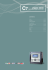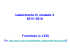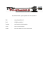# Linear Circuit

## Transcription

Linear Circuit
```Lecture 10
Capacitor and Inductor
Hung-yi Lee
Outline
• Capacitor (Chapter 5.1)
• Inductor (Chapter 5.2)
• Comparison of Capacitor and Inductor
• Superposition for Dynamic Circuits
Outline
• Capacitor (Chapter 5.1)
• Inductor (Chapter 5.2)
• Comparison of Capacitor and Inductor
• Superposition for Dynamic Circuits
Capacitor – i-v characteristics
Dynamic
i (t )
v(t )
dv(t )
i (t )  C
dt
reference current should
flow from “+” to “-”
If v is constant
i=0
Open circuit
1 t
1 t
v(t )   i  d  vt0    i  d
C 
C t0
Capacitor – i-v characteristics
vt 
dv(t )
i (t )  C
dt
Continuity - Capacitor
dv(t )
i (t )  C
dt
• The voltage of capacitor should be continuous
Infinite
current
Current
changes
Capacitor – Power and Energy
Instantaneous consumed
power of a capacitor:
vc (t )
dvc (t )
p (t )  vc (t )  ic (t )  Cvc (t )
dt
Total energy consumed:
ic (t )
dvc t 
E t    pt dt   Cvc t 
dt


dt
p(t )
t
t
1 2
 Cvc (t )
2
The energy stored
Depend on
voltage at t
E(t )
Capacitor - Application
• Automated external defibrillators (AED)
Capacitor - Application
• Automated external defibrillators (AED)
vc (t )  5000
vc (t )  0
5000V
1 2
1
E  Cvc (t )  C  5000 2
2
2
1 2
E  Cvc (t )  0
2
Capacitor - Series
v  v1  v2    v N
dv N
dv1
dv2
i  C1
 C2
   C N
dt
dt
dt
Cser 
1
1
1
1

  
C1 C2
CN
dv d v1  v2    v N 

dt
dt
dv N
dv1 dv2


  
dt
dt
dt
 1
1
1 
  i
  
  
CN 
 C1 C2
1
dv

i
i  Cser
Cser
dt
Capacitor - Parallel
Cpar=C1+C2+ ‥ ‥ ‥+CN
i  i1  i2    iN
dv
dv
dv
 C2
   C N
dt
dt
dt
dv
 C1  C2    C N 
dt
dv
 C par
dt
 C1
Outline
• Capacitor (Chapter 5.1)
• Inductor (Chapter 5.2)
• Comparison of Capacitor and Inductor
• Superposition for Dynamic Circuits
Inductor – i-v characteristics
Dynamic
i (t )
di (t )
v(t )  L
dt
If i=constant
reference current should
flow from “+” to “-”
v=0
v(t )
short circuit
1 t
1 t
i (t )   v d  i t0    v d
L 
L t0
Inductor – i-v characteristics
i t 
di (t )
v(t )  L
dt
Continuity - Inductor
di (t )
v(t )  L
dt
• The current of inductor should be continuous
Infinite
Voltage
Voltage
changes
Shock by Inductor
Inductor – Power and Stored
Energy
• Instantaneous consumed power of an inductor
diL (t )
p ( t )  v L ( t )  iL ( t )  L
 iL ( t )
dt
• Total Energy consumed
diL t 
E t    pt dt   L
iL t dt


dt
t
1 2
 LiL (t )
2
The energy stored
t
Depend on
current at t
Inductor - Series
v  v1  v2    v N
di
di
di
 L1  L2    LN
dt
dt
dt
di
 L1  L2    LN 
dt
di
 Lser
dt
Lser=L1+L2+ ‥ ‥ ‥+LN
Inductor - Parallel
i  i1  i2    iN
diN
di1
di2
v  L1
 L2
   LN
dt
dt
dt
L par 
1
1 1
1
   
L1 L2
LN
di d i1  i2    iN 

dt
dt
diN
di1 di2


  
dt dt
dt
1 1
1 
  v
     
LN 
 L1 L2

1
v
L par
v  L par
di
dt
Outline
• Capacitor (Chapter 5.1)
• Inductor (Chapter 5.2)
• Comparison of Capacitor and Inductor
• Superposition for Dynamic Circuits
Summary - i-v characteristics
dv(t )
i (t )  C
dt

C
V
di (t )
v(t )  L
dt
V
L

Summary - Series and Parallel
Resistor
Capacitor
Inductor
Series
R s   Ri
1
1

Cs
Ci
L s   Li
Parallel
1
1

Rp
Ri
C p   Ci
1
1

Lp
Li
i-v
v(t )  Rit 
dv(t )
i (t )  C
dt
di (t )
v(t )  L
dt
Outline
• Capacitor (Chapter 5.1)
• Inductor (Chapter 5.2)
• Comparison of Capacitor and Inductor
• Superposition for Dynamic Circuits
Review
y   ai xi
This equation only for circuits
with sources and resistors.
i
•
•
y: any current or voltage for an element
xi: current of current sources or voltage of voltage sources
Proportionality Principle, Superposition Principle
Can be used in any circuit in this course
Linearity
• A circuit is a multiple-input multiple-output (MIMO)
system
• Input: current of current sources or voltage of voltage
sources
• Output: the current or voltage for the elements
input
Circuit
(System)
+
v
-
i
output
Linearity
• All linear circuits are linear system
• Linear Circuit:
• Sources
• Linear Elements:
• Resistor, Capacitor, Inductor
v
i
R
All circuits in
this course are
linear circuits.
Linearity
• Linear System:
• Property 1:
Input: g1(t), g2(t), g3(t), ……
output: h1(t), h2(t), h3(t), ……
Input: Kg1(t), Kg2(t), Kg3(t), ……
output: Kh1(t), Kh2(t), Kh3(t), ……
Proportionality Principle
Linearity
• Linear System:
• Property 2:
Input: a1(t), a2(t), a3(t), ……
output: x1(t), x2(t), x3(t), ……
Input: b1(t), b2(t), b3(t), ……
output: y1(t), y2(t), y3(t), ……
Input: a1(t)+ b1(t), a2(t)+ b2(t), a3(t)+ b3(t), ……
output: x1(t)+y1(t), x2(t)+y2(t), x3(t)+y3(t), ……
Superposition Principle
Linearity
• Linear System:
• Property 2:
Input: a1(t), a2(t), a3(t), ……
output: x1(t), x2(t), x3(t), ……
Input: b1(t), b2(t), b3(t), ……
output: y1(t), y2(t), y3(t), ……
Input: a1(t)+ b1(t), a2(t)+ b2(t), a3(t)+ b3(t), ……
output: x1(t)+y1(t), x2(t)+y2(t), x3(t)+y3(t), ……
Superposition Principle
vt 
Linearity
vt 
i t 
g1 t 
0
g t 
g t   g1 t   g 2 t 
Superposition Principle can be
applied on all circuits in this
course (Textbook: Chapter 6.5).
0
i t 
g 2 t 
Announcement
• 10/22 (三) 第一次小考
•
•
•
•
Ch1. Circuit Variables and Laws (1.4, 1.5)
Ch2. Properties of Resistive Circuits (2.3, 2.4, 2.5)
Ch3. Applications of Resistive Circuits (3.2)
Ch4. Systematic Analysis Methods (4.1, 4.2, 4.3, 4.4)
• 助教時間：週一到週四 PM6:30~8:30
• 助教:黃盈庭 [email protected]
• 時間:週三PM6:30~8:30
• 地點:電二146
Thank you!
Appendix
Capacitor – Series (2)
If v is constant
i=0
Open circuit
What are v1, v2 ……?
Capacitor Application
• How Capacitive Liquid Level Sensors Work
Acknowledgement
• 感謝 林楷恩(b02)
• 指出投影片中 Equation 的錯誤
```

### FÂ¡r.t` E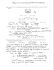### Applications of Magnetism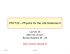### Pagine 127-136 - Tirreno Chapter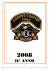### Ventilatore in alluminio Die-cast housing blower Gebläse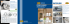### 36kW, 1000Vdc String Inverters for North America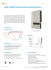### condensatori sistemi e filtri mt e at rifasamento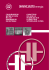### Misura della resistenza d`isolamento e della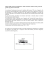### Mike has a dog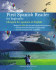### C7 VIMAC 3000